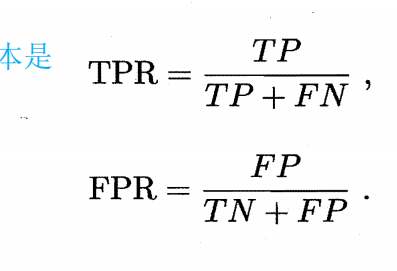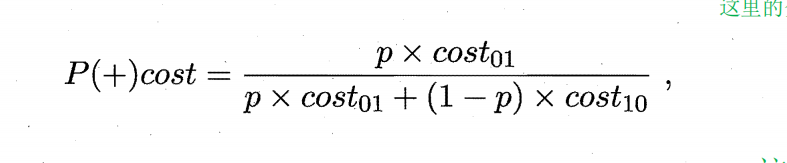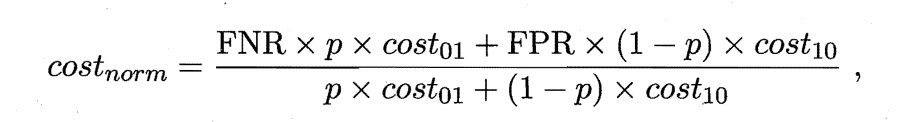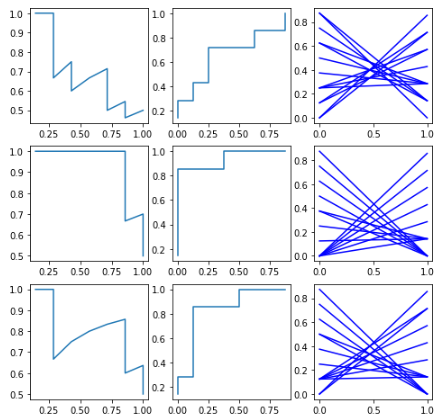• 代价敏感错误率与代价曲线 https://www.bilibili.com/video/BV17J411C7zZ?p=21 视频 import torch import os os.environ["KMP_DUPLICATE_LIB_OK"]="TRUE" output_score = list(range(12)) ## 定义一个列表里面的...
代价敏感错误率与代价曲线
https://www.bilibili.com/video/BV17J411C7zZ?p=21 视频
import torch
import os
os.environ["KMP_DUPLICATE_LIB_OK"]="TRUE"

output_score = list(range(12))  ## 定义一个列表里面的数字是0-11
print(output_score)

y = [0,0,0,0,1,0,1,1,0,1,1,1] ## 其中0表示的是5,1表示的不是5,这里是来对应的标签信息的
print(len(y))
print(y)

# 设定p,集合中正例比列，这里范围是0-100，所以还要除以100
p = list(range(0,101,10)) ## 数字是从0开始，然后所对应步长是10，所以是对应的10个数
p = [i/100 for i in p]   ## 然后这里是转换为0-1之间所对应的数的。
print(p)

# 设定代价
c01 = 3
c02 = 2

#设置判断阈值
theta = 6.5
# 定义函数输出判断
# 我们是来判断是5还是不是5，我们是把打分输入后，和对应界限值theta
def calculate_output_result(output_score,theta):
output_result = []
for i in range(len(output_score)): ## 侧量输出分数所对应的长度的为0 -11
if output_score[i] < theta:  ## 如果小于6.5 则就是0
output_result.append(0)
else:
output_result.append(1) ## 如果是大于6.5则就是1
return output_result
output_result = calculate_output_result(output_score,theta) ## 然后是我们调用这个函数来进行测试
print(output_result)

# 要来统计正例和反例的个数 也就是说其中m+有多少个m-有多少个
import pandas as pd
def calculate_m_positive_negative(y):
result = pd.value_counts(y)
m_positive = result  ## 其中结果是等于1的值是对应的正比例
m_nagative = result  ## 其中对应的0是对应的负的比列的过程
return m_nagative,m_positive
m_positive,m_negative = calculate_m_positive_negative(y)
print(m_positive,m_negative)

# 计算混淆矩阵圈1，圈2，圈3，圈4
def calculate_confusion(y,output_result):
con1 = 0  ## 这里是开始来对混淆矩阵进行初始化的过程的。
con2 = 0
con3 = 0
con4 = 0
for i in range(len(y)):
if y [i] == 1:
if y[i] == output_result[i]:
con1 += 1   ## 其中本来是正例，预测也为正例时所对应的情况的
else:
con2 += 1  ###本来是为正例，但是预测为反例时所对应的情况的
else:
if y[i] == output_result[i]:
con4 += 1   ## 本来是为反列预测也是为反例所对应的情况
else:
con3 += 1   ## 本来为反列，但是预测为正列所对应的情况
return con1,con2,con3,con4
con1,con2,con3,con4 = calculate_confusion(y,output_result)
print(con1,con2,con3,con4)# 求几个数所对应的比列，保留四位小数
def calculate_FNR_FPR(con1,con2,con3,con4):
FNR = round(con2/(con1+con2),4)  ## FNR:表示正例中有多少被预测错了，变成了负例
FPR = round(con3/(con3+con4),4)  ## FPR:表示负列中有多少被预测错了，变成了正例
return FNR,FPR
FNR,FPR = calculate_FNR_FPR(con1,con2,con3,con4)
print(FNR,FPR)# 正概率代价
def calculate_Pcost(p,c01,c02):
Pcosts = []
for i in range(len(p)):   ## 其中我们p是对应的10个不同的值，也就是对应的正样本和负样本所对应的概率的不同，其中每个都会是对应一个层本函数
Pcost = round((p[i]*c01)/(p[i]*c01+(1-p[i])*c02),4)  ## (p[i]*c01+(1-p[i])*c02)这里是来对应的归一化的过程的
Pcosts.append(Pcost)
return Pcosts
Pcosts = calculate_Pcost(p,c01,c02)
print(Pcosts)# 归一化总概率 其中p是对应的不同的正负样本所对应的概率的。
def calculate_cost_norm(p,c01,c02,FNR,FPR):
costs_norm = []
for i in range(len(p)):
cost_norm = round((FNR*(p[i]*c01)+FPR*(1-p[i])*c02)/(p[i]*c01+(1-p[i])*c02),4)
costs_norm.append(cost_norm)
return costs_norm
costs_norm = calculate_cost_norm(p,c01,c02,FNR,FPR)
print(costs_norm)  ## 是在p不断的变化的时候每一个p所对应的总的归一化的所对应的概率的。

## 我们来画出图像
import matplotlib.pyplot as plt
def plot_lines(X,Y,color):  ## 首先是来定义的函数的，然后再来进行相应的调用的过程的。
plt.plot(X,Y,color)
return
plot_lines(Pcosts,costs_norm,'r')
plot_lines(p,costs_norm,"b:")
plt.show()

## 这里前面的是对应的一个theta所对应的情况的

## 后面我们是对应的多个theta所对应的情况的

# 生成多个theta
thetas = list(range(12))
thetas = [i+0.5 for i in thetas]  ## 这里的thetas值是从0.5开始的
print(thetas)   ## 这里是来的到多个theta的是，也就是是所对应的权限的值的过程的。

#定义计算每个theta对应的点的函数，并且存放在列表中
# output_score是输出相应的分数的值，
# y是对应的真是的标签
# calculate_Pcost 正概率所对应的代价
# calculate_cost_norm:归一化总的概率
def calculate_Pcost_cost_norm(thetas,output_socre,y,calculate_Pcost,calculate_cost_norm):
Pcosts_n = []
costs_norm_n = []
theta_FPR_FNR = []
for i in range(len(thetas)):
theta = thetas[i]  ## 这里是来得到每个theta所对应的值

# 计算输出结果，通过theta来对应的边界，这样就是能够通过相应输入分来标签进行预测
output_result = calculate_output_result(output_score,theta)
# print(output_result)  ## 这个预测值是不止一个值的

# 统计正反例个数  通过样本的标签来统计正例和反列所对应的个数的。
m_positive,m_negative = calculate_m_positive_negative(y)

# 计算混淆矩阵
con1,con2,con3,con4 = calculate_confusion(y,output_result)
# print(con1,con2,con3,con4)

# 求FNR,FPR，其中FNR有多少正样本被预测错了，变成了反例，FPR是表示负例样本中有多少被预测错了，变成了正例
FNR,FPR = calculate_FNR_FPR(con1,con2,con3,con4)
theta_FPR_FNR[theta]:[FNR,FPR]  ## 通过列表的形式把这这些给存储起来，其中每一个theta都是对应一对[FNR,FRP]所
## 对应的值过程的。

#正概率代价  # 这里是来对应的不同的概率的过程
Pcosts = calculate_Pcost(p,c01,c02)
Pcosts_n.append(Pcosts)

# 归一化总概率 # 这里也是对应的不同的概率的过程的。
costs_norm = calculate_cost_norm(p,c01,c02,FNR,FPR)
costs_norm_n.append(costs_norm)

return Pcosts_n,costs_norm_n,theta_FPR_FNR  ## 其中是每一个theta都是对应一条线，（FNR,FPR）就是对应一条线，
## 然后又是对应的不同的概率的，
# 调用这个函数来进行计算
Pcosts_n,costs_norm_n,theta_FPR_FNR = calculate_Pcost_cost_norm(thetas,output_score,y,calculate_Pcost,calculate_cost_norm)

## 这里是来画出线段的过程的。
for i in range(len(Pcosts_n)):  ## 这里总共是可以得到12条线段的。
plot_lines(Pcosts_n[i],costs_norm_n[i],'r')
plt.show()



展开全文机器学习
• 代价曲线代价Yes, it compiled. It worked even. Not only did it work, but it completely meets the client's requirements. But somehow when I look at those particular lines of code (16 lines, in this case...代价曲线代价Yes, it compiled.  It worked even.  Not only did it work, but it completely meets the client's requirements.  But somehow when I look at those particular lines of code (16 lines, in this case, with DataGridGirl's help) I wonder about the ramifications of this horrible algorithm on my immortal soul.
是的，它已编译。 它甚至工作。 它不仅有效，而且完全可以满足客户的要求。 但是不知何故，当我查看那些特定的代码行(在本例中为16行，在DataGridGirl的帮助下)时，我想知道这种可怕算法对我不朽灵魂的影响。
If you know me, or maybe even if you don't, you know that it has been said I have an overdeveloped sense of smell.  Specifically, Code Smell.  Often it's a gift and has served me well.  Other times it's a crushing weight as the schedule looms and I just have to buck and, well, write some crap.  (Of course, if it is totally with in spec, performance, it works, QA, etc, it's not crap, but you know.)
如果您了解我，或者即使您不了解，您也知道有人说我的嗅觉过高。 具体来说，代码气味。 通常这是一份礼物，对我很有帮助。 其他时候，由于日程安排迫在眉睫，这真是沉重的负担，我只好反击，写些废话。 (当然，如果它在规格，性能，质量，质量保证等方面完全符合要求，那不是废话，但您知道。)
So my question to you, fair readers and fellow codesmith's, how bad do you feel when the pattern doesn't quite fit or that nested foreach/foreach/foreach nested a bit to deep?
因此，我想向您提出的问题是，公平的读者和同为Codesmith的人，当模式不太合适或嵌套的foreach / foreach / foreach嵌套得很深时，您感觉如何？
When do you know to let go? Some might say, “when it passes the NUnit Test!”  Others, who look at coding as much an art form as science might disagree.
你什么时候知道放手有人可能会说：“当它通过NUnit测试时！” 其他人则认为编码和科学一样多的艺术形式可能会不同意。
I noticed a number of folks, from Don Box to Doug Purdy, at the PDC making GREAT PAINS to let the audience know that “this or that particular line of code is an abomination! Look away, look away from these alpha bits that hold all our pride and all our shame.”  I had to say I agreed with their evaluations.
我注意到在PDC上有很多人，从Don Box到Doug Purdy ，都在制作GREAT PAINS，以使观众知道“这一行或那一行特定的代码真是令人讨厌！ 移开视线，远离保持我们所有自豪感和羞辱感的这些alpha位。 我不得不说我同意他们的评价。
What to do when you've built a great monument, but there's bird poop at the top that you're responsible for.  Does it take away from the accomplishment?

当您建造了一座宏伟的纪念碑时该怎么办，但是您要负责的是顶部的便便。 它会剥夺成就吗？翻译自: https://www.hanselman.com/blog/but-what-price-my-soul代价曲线代价
展开全文机器学习 php webgl stm32 google
• 举例说明 P-R图的计算 ROC曲线的计算 代价曲线的计算与绘制 以上三者都要求逐个把样本作为正例进行预测，模拟的是threshold设为不同值时同一个模型的预测效果 原理内容请参考《机器学习》周志华 2.3节。也可查看...
举例说明  P-R图的计算          ROC曲线的计算          代价曲线的计算与绘制
以上三者都要求逐个把样本作为正例进行预测，模拟的是threshold设为不同值时同一个模型的预测效果
原理内容请参考《机器学习》周志华  2.3节。也可查看以下网址

PR曲线和F1、ROC曲线和AUC

模型评估与选择（后篇）-代价曲线
只需要在下面的模块中更改model_sequence里面的分类序列的顺序。以下为针对某一二分类问题的三个模型的P-R图、ROC曲线、代价曲线。

P-R图，如果模型A的P-R 图将模型B的P-R图完全包住，则A效果比B好。同时还要看BEP和F1值也可以用来比较模型效果。

ROC曲线，曲线下方面积为AUC，AUC越大，模型效果越好。曲线上方部分面积为loss，loss越小模型越好。模型A的ROC曲线如果完全把模型B的ROC曲线包在下方则模型A的效果比B好。如果两者并不是完全包含与被包含的关系，而是有重叠区域也有未重叠区域，则用AUC值比较。

代价曲线是在出现非平衡代价的情况下才使用的。所有曲线最下方的面积越小，则代表模型越好。

如下9幅图为3个模型对同一个样本的分类结果。第一行为model_1的P-R图、ROC曲线、代价曲线。第二行为model_2,第三行为model_3。三个model对比下来model_2效果最好。绘制过程见下文代码。# 假设我们的样本是7个1和8个0.    1作为正例，0作为负例
model_1 = [1,1,0,1,0,1,1,0,0,0,1,0,0,1,0]
model_2 = [1,1,1,1,1,1,0,0,0,1,0,0,0,0,0]
model_3 = [1,1,0,1,1,1,1,0,0,0,1,0,0,0,0]
model_sequence = [model_1,model_2,model_3]

def PNTF(model_sequence, n):# 将第几个样本之前的全部作为正例。n是从1开始的，不是从0开始的
count = len(model_sequence)
class_1_count = sum(model_sequence)
class_0_count = count - class_1_count
if (n>count - 1) or (n<1):
return None
else:
TP = sum(model_sequence[0:n])
FN = class_1_count - TP
FP = n - TP
TN = class_0_count - FP
return TP,FN,TN,FP

def P_R(TP,FN,TN,FP):
P = TP/(TP+FP)
R = TP/(TP+FN)
F1 = 2*P*R/(P+R)
return P,R,F1

def ROC_TPR_FPR(TP,FN,TN,FP):
TPR = TP/(TP+FN)
FPR = FP/(TN+FP)
return TPR,FPR

def cost_curve_FPR_FNR(TP,FN,TN,FP):
TPR = TP/(TP+FN)
FNR = 1- TPR#假反率
FPR = FP/(TN+FP)
return FPR,FNR

#只是用于test
def PR_ROC_Cost_Curve_public_part(model_sequence,verbose = False):
length = len(model_sequence)
if verbose==True:
print('把前n个样本作为正例进行预测')
print('n\t','TP\t','FN\t','TN\t','FP\t','P\t','R\t','F1\t','TPR\t','FPR\t','FNR')
list_P_R_F1 = []
list_ROC_TPR_FPR = []
list_cost_curve_FPR_FNR = []
for i in range(length - 1):
TP,FN,TN,FP = PNTF(model_sequence,i+1)
P,R,F1 = P_R(TP,FN,TN,FP)
TPR,FPR = ROC_TPR_FPR(TP,FN,TN,FP)
FPR,FNR = cost_curve_FPR_FNR(TP,FN,TN,FP)
if verbose ==True:
print(i+1,'\t',TP,'\t',FN,'\t',TN,'\t',FP,'\t',round(P,3),'\t',round(R,3),'\t',round(F1,3),'\t',round(TPR,3),'\t',FPR,'\t',round(FNR,3))
list_P_R_F1.append([P,R,F1])
list_ROC_TPR_FPR.append([TPR,FPR])
list_cost_curve_FPR_FNR.append([FPR,FNR])
return list_P_R_F1,list_ROC_TPR_FPR,list_cost_curve_FPR_FNR

import matplotlib.pyplot as plt
import numpy as np
model_count = len(model_sequence)
fig,axis = plt.subplots(model_count,3,figsize = (8,8))
axis = axis.ravel()
count = 0
for i in range(model_count):
list_P_R_F1,list_ROC_TPR_FPR,list_cost_curve_FPR_FNR = PR_ROC_Cost_Curve_public_part(model_sequence[i])
array_P_R_F1 = np.array(list_P_R_F1)
P = array_P_R_F1[:,0]
R = array_P_R_F1[:,1]
axis[count].plot(R,P)
count +=1

array_ROC_TPR_FPR = np.array(list_ROC_TPR_FPR)
TPR = array_ROC_TPR_FPR[:,0]
FPR = array_ROC_TPR_FPR[:,1]
axis[count].plot(FPR,TPR)
count +=1

array_cost_curve_FPR_FNR = np.array(list_cost_curve_FPR_FNR)
FPR = array_cost_curve_FPR_FNR[:,0]
FNR = array_cost_curve_FPR_FNR[:,1]
for j in range(len(FPR)):
axis[count].plot((0,1),(FPR[j],FNR[j]),'b')
count +=1


展开全文机器学习 ROC
• 机器学习（周志华）第2.3.4节中，代价曲线的理解？ - xf3227的回答 - 知乎 https://www.zhihu.com/question/63492375/answer/247885093 模型评估与选择（后篇）-代价曲线 文章目录基本概念定义：代价直线（未归一化...
• 在这片博客里我将记录模型评价ROC曲线以及AUC面积的概念以及作用，并且同样以二分类任务为例介绍一个新的模型评价标准，那就是代价曲线 ROC于AUC 在这里我们要引入2个新的概念，那就是真正例率（True Positive Rate...机器学习
• 代价敏感错误率 代价曲线 转载于:https://www.cnblogs.com/ForTech/p/8548883.html
• 性能度量——代价曲线一、常见的性能度量1、错误率/精度2、precision/recall 及F13、ROC/AUC二、常见的性能度量方式的优缺点1、单标量（错误率，精度，auc）：2、ROC：三、Cost Curves1、前导2、均等代价时，从ROC ...
• 看博客上都在说书上关于代价曲线的介绍太过于简单，只考书中的内容无法理解，我当时看完竟然咩有这种感觉，看来功力是完完全全的不够啊！建议大家看原论文（有30多页，我没去看，有时间的朋友可以阅读一下） 知乎...机器学习
• 代价曲线书中关于代价曲线介绍的太简洁了，只靠书中的内容是无法理解的。建议大家去看原论文。从分类器的ROC曲线上无法看出分类器的期望代价，而代价曲线是专门用于度量期望代价和operating condition之间的关系的。...机器学习
• 我们根据学习器的预测结果对样例进行排序，按此顺序逐个把样本作为正例进行预测，每次计算出两个重要量的值，与P—R曲线的使用查准率与查全率为纵，横轴不同，ROC曲线的纵轴是“真正例率(TPR，True Positive Rate)”...列表 python 机器学习 深度学习 人工智能
• 上文详细介绍了P-R曲线与ROC曲线的性能评估方法，P-R曲线与ROC曲线是基于均等损失代价的模型性能评估方法，本文承接上文，详细介绍基于非均等损失代价的模型性能评估方法，并对已介绍的性能评估方法进行了总结。...
• 转发：https://blog.csdn.net/algorithmpro/article/details/83870768 具体内容请参考链接 ，文章写的很好，关于机器学习的性能评估方法！机器学习 性能评估
• 下一篇文章将介绍另一种评估分类器的曲线”代价曲线“，它在一定程度上解决了这两个问题，并与ROC曲线形成了良好的互补。 参考文献 Tom Fawcett, An introduction to ROC analysis, Pattern Recognition Letters, 27...机器学习
• https://www.zhihu.com/question/63492375
• https://www.zhihu.com/question/63492375
• 在知乎上看到了很好的回答，直接附上链接link~ 由于怕之后找不到，干脆再复制粘贴一份~机器学习
• 2.理解：ROC主要考量均等代价代价敏感曲线主要考量非均等代价。两者都是衡量某一学习器在不同场景下的综合表现情况，而不是单一场景。ROC通过阈值变化来体现不同场景，即高阈值表现了重视查准率的场景，低阈值则...
• P-R曲线 ROC曲线与AUC 代价(CC)曲线python 机器学习
• 以线性回归多项式为例，探索代价函数与训练数据集大小的关系。 %matplotlib inline import matplotlib.pyplot as plt import numpy as np n_dots = 200 # 创建数据集y=sqrt(x) X=np.linspace(0,1,n_dots) y=np....机器学习
• 标红线的地方，是比较巧妙的地方，梯度下降法的过程中，存储了每次迭代得到的代价函数，就可以画出代价函数关于迭代次数的学习曲线。 详情，可以参考Andrew Ng couresa machine learning的课程week2 联系提供的...
• 回顾线性回归的正则化1.1 代价函数1.2 梯度2. 偏差与方差2.1 训练集数量对“代价”的影响3. 多项式回归3.1 多项式特征的生成3.2 lambda对“代价”的影响 照旧，本练习的相关资料链接将会扔到评论区，大家自取 1. ......